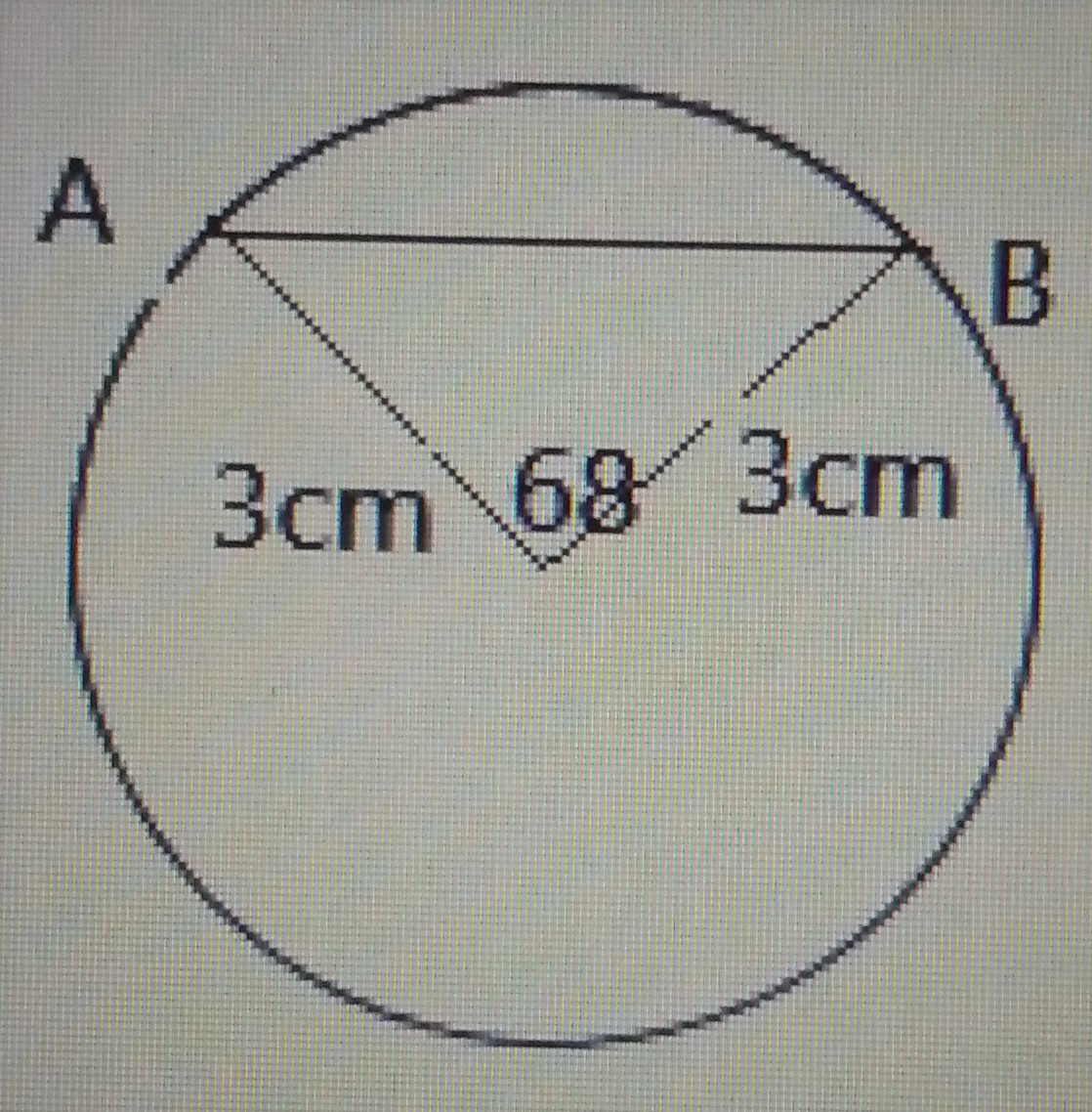# Mathematics 2019 Past Questions | JAMB

Study the following Mathematics past questions and answers for JAMBWAEC  NECO and Post-JAMB. Get prepared with official past questions and answers for upcoming examinations.

1. Make q the subject of the formula in the equation $$\frac{mn}{a^2} – \frac{pq}{b^2} = 1$$
• A.  $$q = \frac{b^2(mn – a^2)}{a^2 p}$$
• B.  $$q = \frac{m^2 n – a^2}{p^2}$$
• C.  $$q = \frac{mn – 2b^2}{a^2}$$
• D.  $$q = \frac{b^2 (n^2 – ma^2)}{n}$$

2.  The angle of elevation of the top of a tree from a point on the ground 60m away from the foot of the tree is 78°. Find the height of the tree correct to the nearest whole number.

• A. 148m
• B. 382m
• C. 282m
• D. 248m

3.  A binary operation  is defined by mn=mn+mnm⊗n=mn+m−n on the set of real numbers, for all m, n  R. Find the value of 3  (2  4).

• A. 6
• B. 25
• C. 15
• D. 18
4.
 Age in years 7 8 9 10 11 No of pupils 4 13 30 44 9
The table above shows the number of pupils in a class with respect to their ages. If a pie chart is constructed to represent the age, the angle corresponding to 8 years old is
• A. 48.6°
• B. 56.3°
• C. 46.8°
• D. 13°

5.  In a class of 50 students, 40 students offered Physics and 30 offered Biology. How many offered both Physics and Biology?

• A. 42
• B. 20
• C. 70
• D. 54
6. Rationalize  $$\frac{\sqrt{2} + \sqrt{3}}{\sqrt{2} – \sqrt{3}}$$
• A. $$-5 – 2\sqrt{6}$$
• B. $$-5 + 3\sqrt{2}$$
• C. $$5 – 2\sqrt{3}$$
• D. $$5 + 2\sqrt{6}$$

7.Find the length of the chord |AB| in the diagram shown above.

• A. 4.2 cm
• B. 4.3 cm
• C. 3.2 cm
• D. 3.4 cm

8. Given  $\mathrm{sin}58°=\mathrm{cos}p°$

find p.

• A. 48°
• B. 58°
• C. 32°
• D. 52°

9. $$\frac{\frac{2}{3} \div \frac{4}{5}}{\frac{1}{4} + \frac{3}{5} – \frac{1}{3}}$$

• A. $$\frac{31}{50}$$
• B. $$\frac{20}{31}$$
• C. $$\frac{31}{20}$$
• D. $$\frac{50}{31}$$

10. If $$6x^3 + 2x^2 – 5x + 1$$ divides $$x^2 – x – 1$$, find the remainder.

• A. 9x + 9
• A. 2x + 6
• B. 6x + 8
• C. 5x – 3

Scroll to Top